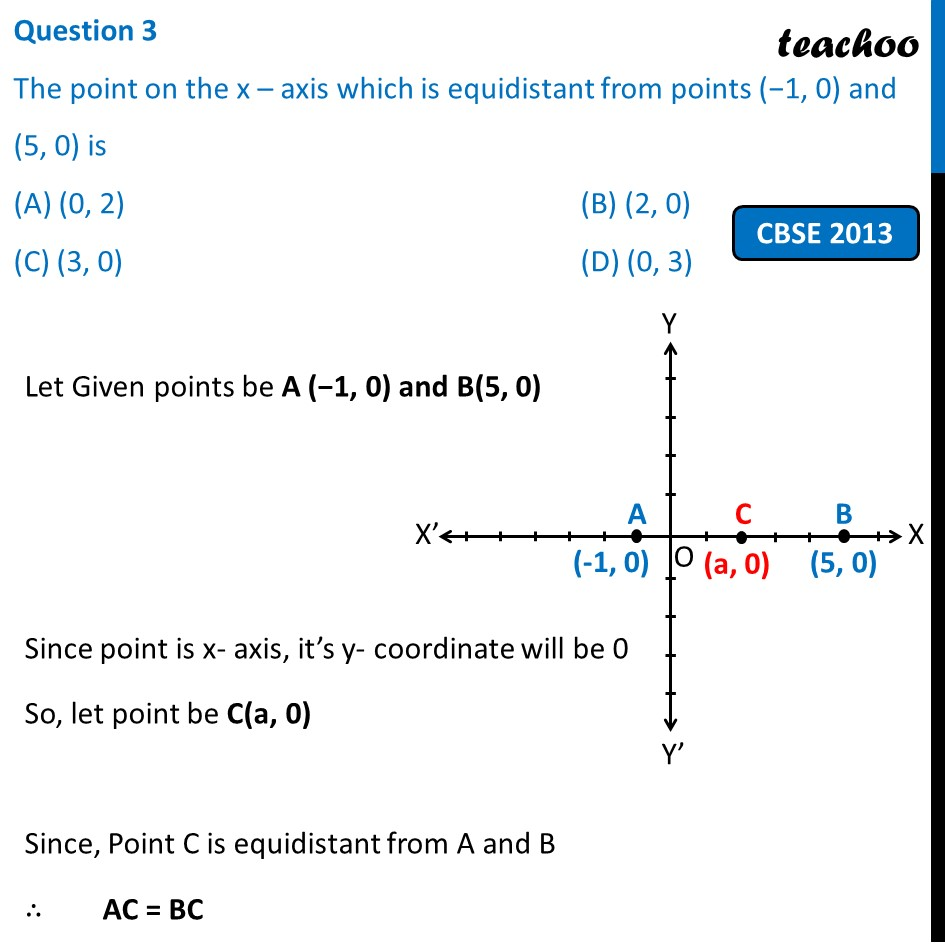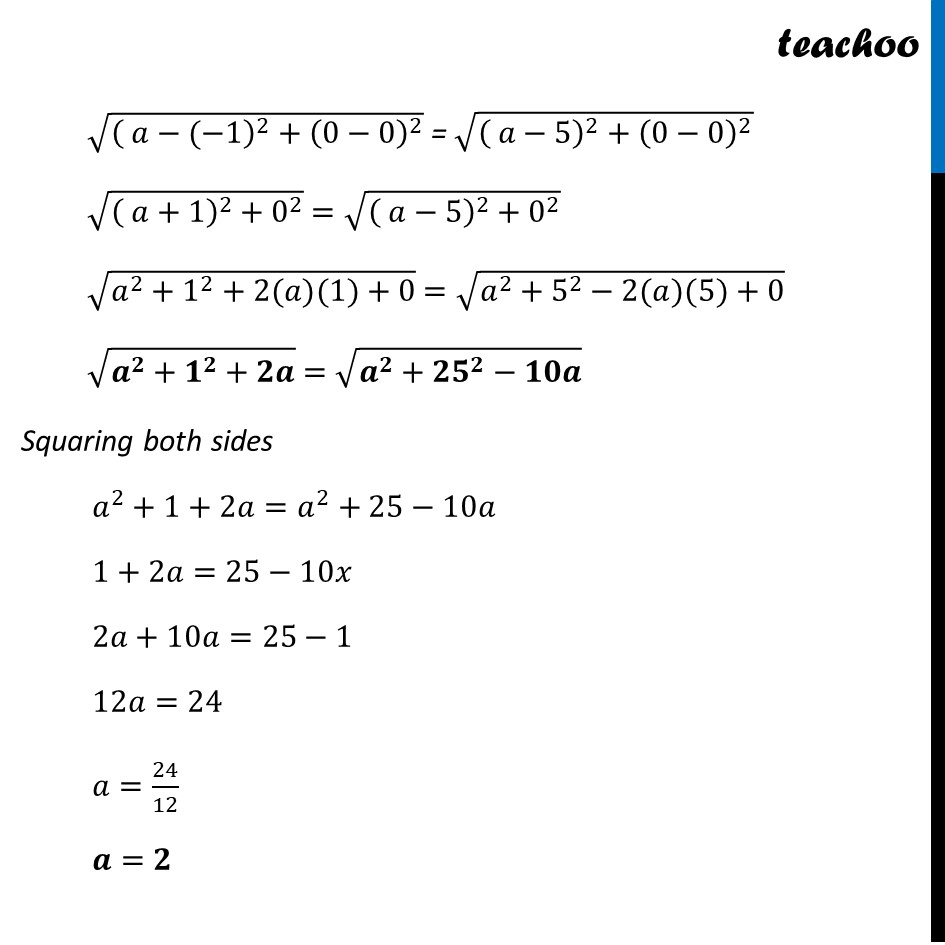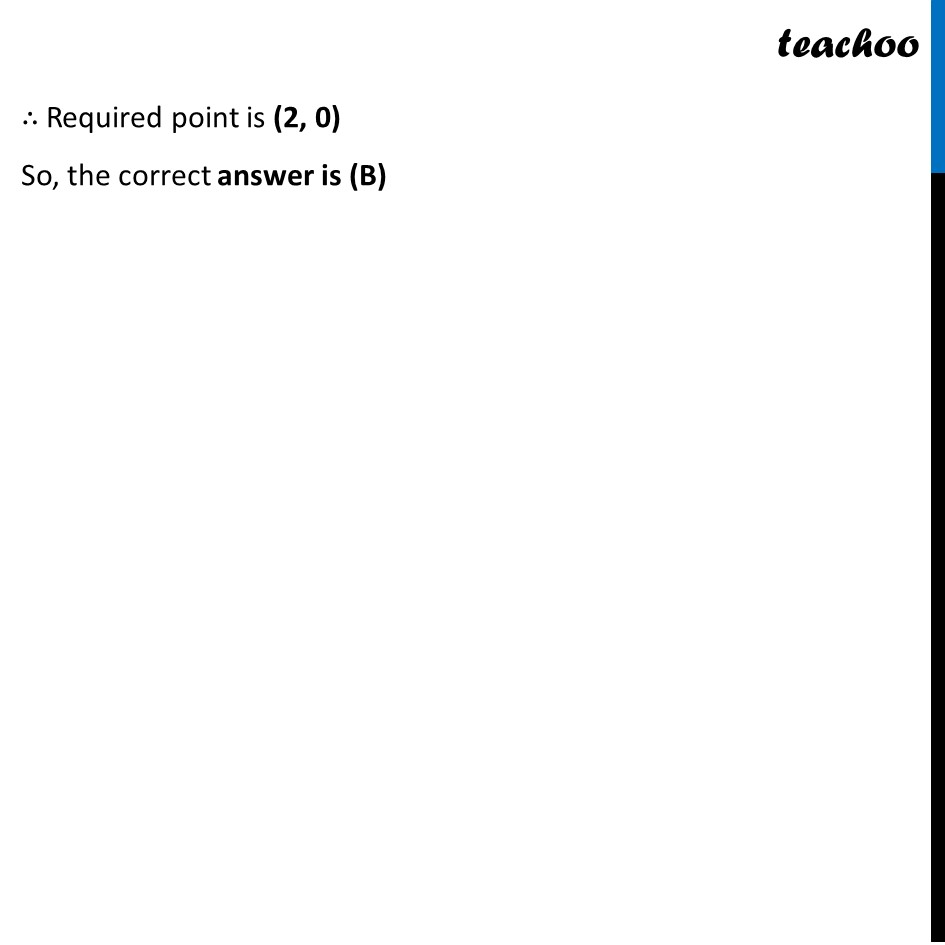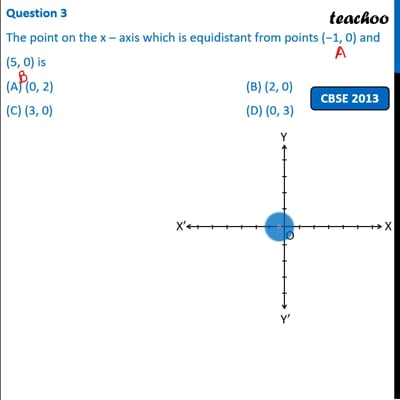Past Year MCQ

Chapter 7 Class 10 Coordinate Geometry (Term 1)
Serial order wise

## D) (0, 3)

This question is similar to Ex 7.1, 7 - Chapter 7 Class 10 Coordinate GeometryThis video is only available for Teachoo black users

### Transcript

Question 3 The point on the x – axis which is equidistant from points (−1, 0) and (5, 0) is (A) (0, 2) (B) (2, 0) (C) (3, 0) (D) (0, 3) Let Given points be A (−1, 0) and B(5, 0) Since point is x- axis, it’s y- coordinate will be 0 So, let point be C(a, 0) Since, Point C is equidistant from A and B ∴ AC = BC √(( 𝑎−(−1)^2+(0−0)^2 ) = √(( 𝑎−5)^2+(0−0)^2 ) √(( 𝑎+1)^2+0^2 ) =√(( 𝑎−5)^2+0^2 ) √(𝑎^2+1^2+2(𝑎)(1)+0) =√(𝑎^2+5^2−2(𝑎)(5)+0) √(𝒂^𝟐+𝟏^𝟐+𝟐𝒂) =√(𝒂^𝟐+〖𝟐𝟓〗^𝟐−𝟏𝟎𝒂) Squaring both sides 𝑎^2+1+2𝑎=𝑎^2+25−10𝑎 1+2𝑎=25−10𝑥 2𝑎+10𝑎=25−1 12𝑎=24 𝑎=24/12 𝒂=𝟐 ∴ Required point is (2, 0) So, the correct answer is (B)